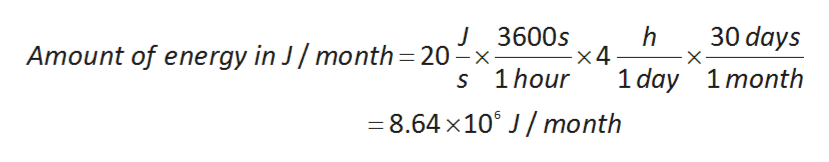# The energy required to power a light bulb is 20 W . On an average, the bulb is switched on for 4 hours per day. Calculate the amount of energy required in joules per month? Assume 30 days in one month.

Question
4 views

The energy required to power a light bulb is 20 W . On an average, the bulb is switched on for 4 hours per day. Calculate the amount of energy required in joules per month? Assume 30 days in one month.

check_circle

star
star
star
star
star
1 Rating
Step 1

The concept is used to solve the problem is forms of energy and energy conversion factors.

The capacity to do a work is called as the energy.

Eelctrical energy is the from of energy and it is created by flow of electrical charge.

The conversion factor of energy from watt to joule per second is as follows:

Step 2

The energy required to power a light is .

Convert the amount of energy required to power a light from watts to joules per second as follows:

Step 3

The amount of energy in joule per second is 20 J/s.

In one day, the light is switched on for 4 h .

Calculate the amount of energy required in jole per month as follows:

...help_outlineImage TranscriptioncloseJ 3600s x4 S 1hour 30 days h Amount of energy in J/ month 20 x- 1 day 1month 8.64 x10 J/month fullscreen

### Want to see the full answer?

See Solution

#### Want to see this answer and more?

Solutions are written by subject experts who are available 24/7. Questions are typically answered within 1 hour.*

See Solution
*Response times may vary by subject and question.
Tagged in

### Chemistry